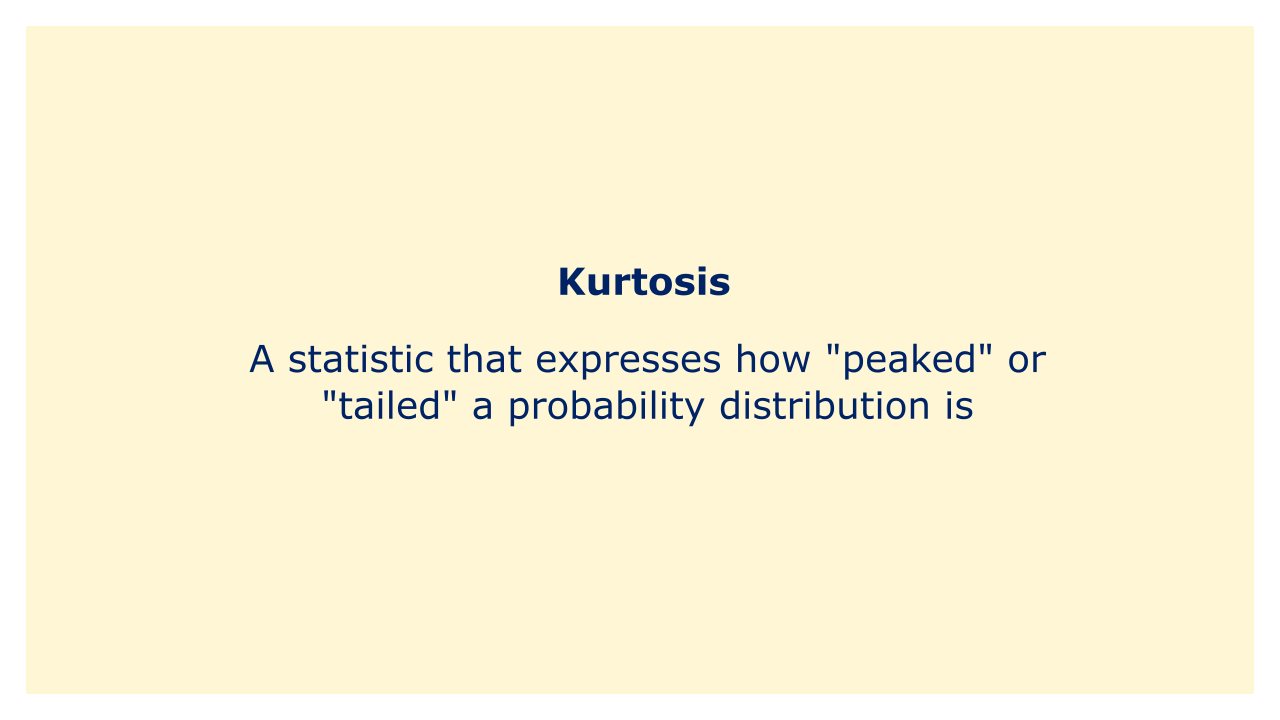# KurtosisImage: Moneybestpal.com

### Kurtosis is a statistic that expresses how "peaked" or "tailed" a probability distribution is. It is a number that expresses how much of the data, in relation to the distribution's tails, is concentrated around the distribution's mean. Kurtosis, specifically, gauges the distribution's tails' degree of curvature in relation to the normal distribution.

The tails of the distribution are not more or less peaked than the tails of a normal distribution because a normal distribution has a kurtosis of zero. Whereas a distribution with a negative kurtosis has tails that are less peaked or lighter than a normal distribution, one with a positive kurtosis has heavier or more peaked tails than the other.

Excess kurtosis and standardized kurtosis are two examples of the many various ways that kurtosis can be measured. The excess kurtosis, which is calculated as the kurtosis minus 3, calculates how far from a normal distribution the distribution deviates. For comparing kurtosis values for various sample sizes and distributions, the standardized kurtosis, which is the excess kurtosis divided by the kurtosis' standard error, is employed.

Kurtosis is a crucial indicator of a probability distribution's shape since it tells us how concentrated and dispersed the data are. While a high kurtosis value suggests that the distribution of returns is more heavily tailed and hence has a higher possibility of extreme occurrences or outliers, kurtosis is frequently used in finance to measure the risk of investment portfolios.
Tags